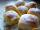Several pieces of bread were in the store. Half of them plus a half of bread bought a cookbook. Of the remaining bread, half of it plus half of the bread was bought by Mr. Novák. The last bread was bought by Ms. Small. How many breads were in the store at the beginning?

Result

n =  7

Solution:

(n/2 + 1/2) + (n-(n/2 + 1/2))/2 + 1/2 + 1 = n

0.5n = 3.5

n = 7

Calculated by our simple equation calculator.

Leave us a comment of this math problem and its solution (i.e. if it is still somewhat unclear...):Be the first to comment!To solve this verbal math problem are needed these knowledge from mathematics:

Need help calculate sum, simplify or multiply fractions? Try our fraction calculator. Do you have a linear equation or system of equations and looking for its solution? Or do you have quadratic equation?

Next similar math problems:

1. CakesOn the bowl were a few cakes. Jane ate one-third of them, Dana ate a quarter of those cakes that remained. a) What part (of the original number of cakes) Dana ate? b) At least how many cakes could be (initially) on thebowl?
2. Fraction and a decimalWrite as a fraction and a decimal. One and two plus three and five hundredths
3. Simple equation 5Solve equation with fractions: X × 3/8 = 1/2
4. ZdeněkZdeněk picked up 15 l of water from a 100-liter full-water barrel. Write a fraction of what part of Zdeněk's water he picked.
5. Eq1Solve equation: 4(a-3)=3(2a-5)
6. Lengths of the poolMiguel swam 6 lengths of the pool. Mat swam 3 times as far as Miguel. Lionel swam 1/3 as far as Miguel. How many lengths did mat swim?Mom bake cookies. Rolo took 2/9 of all cookies, Michal 3/9. How many cookies ate Rolo if Michal had 9.
8. Six te 2If 3t-7=5t, then 6t=
9. Addition of Roman numbersAdded together and write as decimal number: LXVII + MLXIV
10. CagesHonza had three cages (black, silver, gold) and three animals (guinea pig, rat and puppy). There was one animal in each cage. The golden cage stood to the left of the black cage. The silver cage stood on the right of the guinea pig cage. The rat was in the
11. PopsiclesFrancis went to buy ice lollies. If he buy 8 popsicles he missed 4 USD. When he buy 7 popsicles, got back 1 USD. How many USD was a popsicle?
12. Jam cakesMom baked a third of plum jam cakes, one third cheesecakes and 18 poppy. How many cakes she had bake?
13. In fractionsAn ant climbs 2/5 of the pole on the first hour and climbs 1/4 of the pole on the next hour. What part of the pole does the ant climb in two hours?
14. Equation 20In given equation: 8/9-4/5=2/9+x, find x
15. Simple equation 8Solve the following equation: 36=-(1+7x)-6(-7-x)
16. Mixed2improperWrite the mixed number as an improper fraction. 166 2/3
17. Fraction to decimalWrite the fraction 3/22 as a decimal.